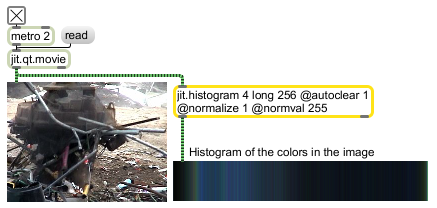# jit.histogram

Calculate histogram

## Description

The jit.histogram object calculates the histogram for a series of input matrices. The histogram is the distribution of values for each of the matrix's planes.

## Matrix Operator

matrix inputs:1, matrix outputs:1
 Name IOProc Planelink Typelink Dimlink Plane Dim Type out n/a 1 0 0 1 1 long char

## Attributes

Name Type g/s Description
autoclear int Autoclear flag (default = 1) When the flag is set, the matrix is cleared following output.
normalize int Normalization mode. The normalization modes are:

0: no normalization
1: per channel normalization
Channel-linked normalization may be of limited value for video signals where the alpha channel is composed of all zero values.
normval int Normalization flag (default = 0) When the flag is set, histogram values to the range [0, normval ] are normalized.

## Examples